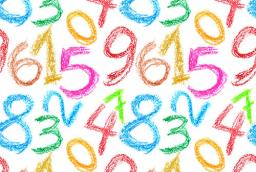# Numbers 3053

The sum of the two numbers is 98. The first number is 4/3 times larger than the second. What are the numbers?

a =  56
b =  42

### Step-by-step explanation:

a+b=98
a = 4/3·b

a+b = 98
3a-4b = 0

Pivot: Row 1 ↔ Row 2
3a-4b = 0
a+b = 98

Row 2 - 1/3 · Row 1 → Row 2
3a-4b = 0
2.33b = 98

b = 98/2.33333333 = 42
a = 0+4b/3 = 0+4 · 42/3 = 56

a = 56
b = 42

Our linear equations calculator calculates it.Did you find an error or inaccuracy? Feel free to write us. Thank you!

Tips for related online calculators
Do you have a linear equation or system of equations and looking for its solution? Or do you have a quadratic equation?